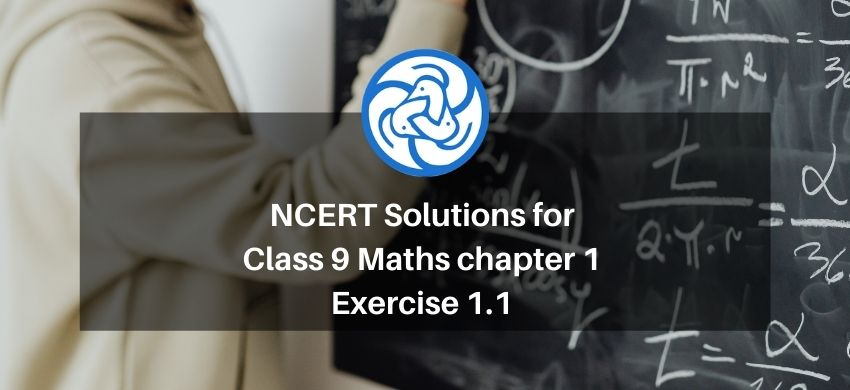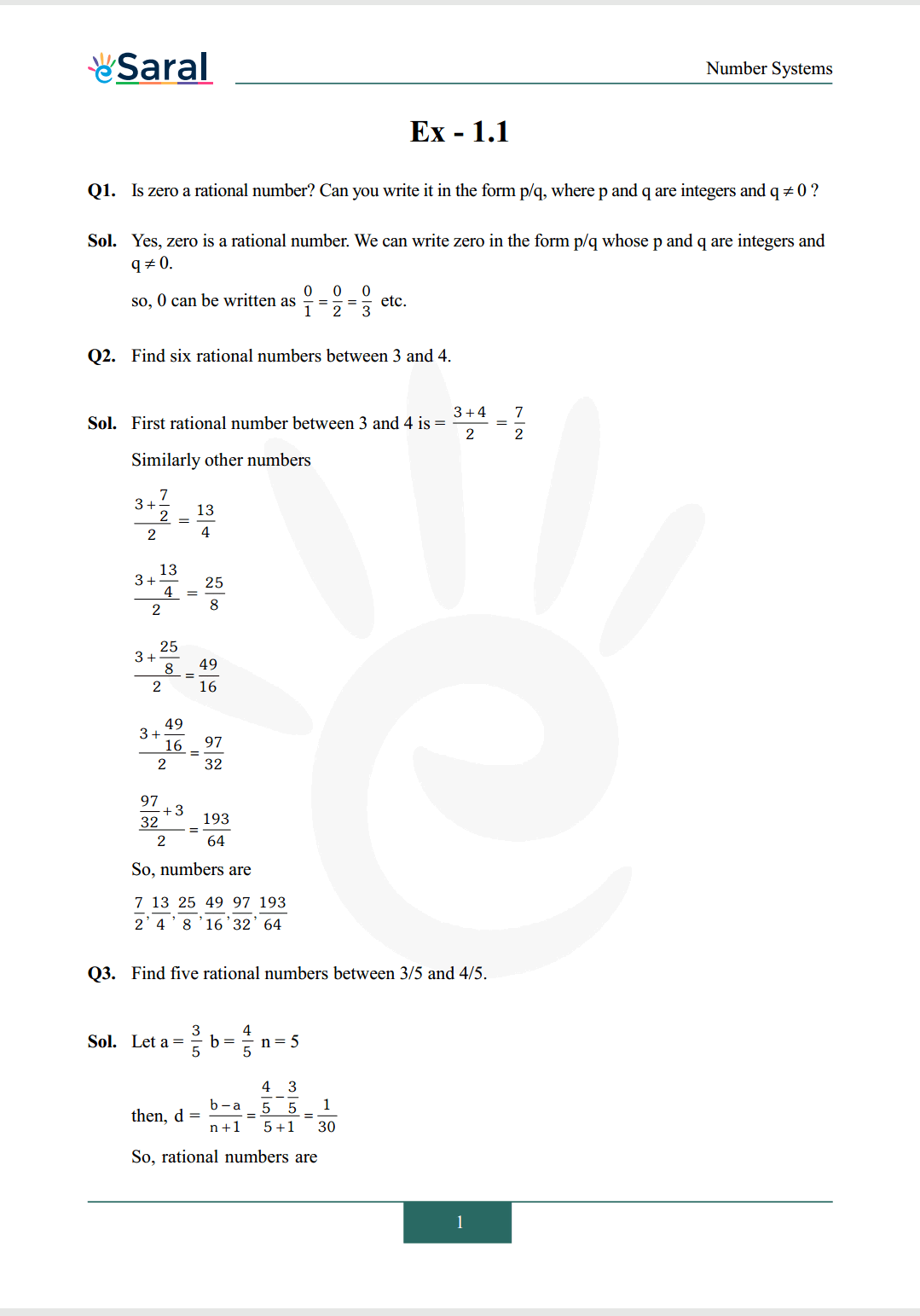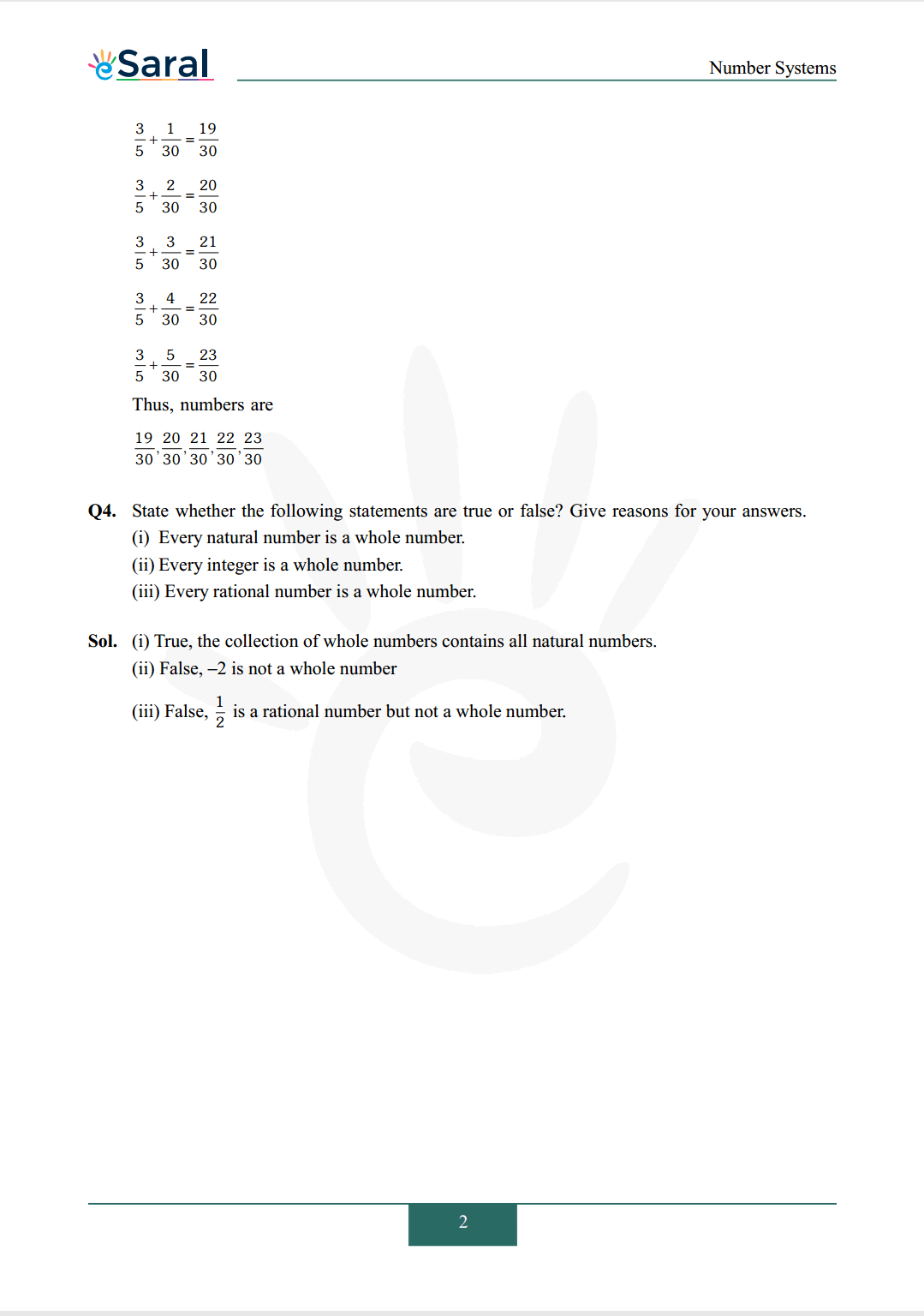# NCERT Solutions for Class 9 Maths chapter 1 Exercise 1.1 - Number System - Free PDF DownloadHey, are you a class 9 Student and Looking for Ways to Download NCERT Solutions for Class 9 Maths chapter 1 Exercise 1.1? If Yes then you are at the right place.

Here we have listed Class 9 maths chapter 1 exercise 1.1 solutions  in PDF that is prepared by Kota’s top IITian’s Faculties by keeping Simplicity in mind.

If you want to score high in your class 9 Maths Exam then it is very important for you to have a good knowledge of all the important topics, so to learn and practice those topics you can use eSaral NCERT Solutions.

So, without wasting more time Let’s start.

### Download The PDF of NCERT Solutions for Class 9 Maths chapter 1 Exercise 1.1 "Number System"#### All Questions of Chapter 1 Exercise 1.1

Once you learned the chapter 1 Exercise 1.1 then you can revise the Exercise by solving these questions.

Q1.   Is zero a rational number? Can you write it in the form p/q, where p and q are integers and?

Q2.   Find six rational numbers between 3 and 4.

Q3.   Find five rational numbers between 3/5 and 4/5.

Q4.   State whether the following statements are true or false? Give reasons for your answers.
(i)  Every natural number is a whole number.
(ii) Every integer is a whole number.
(iii) Every rational number is a whole number.

Complete Class 9 Maths Short Revision Notes.

Class 9 NCERT Maths Book PDF

Class 9 NCERT Maths Exemplar

Complete Solutions for Class 9 chapter 1 Number Systems

If you have any Confusion related to NCERT Solutions for Class 9 Math chapter 1 Exercise 1.1 then feel free to ask in the comments section down below.

To watch Free Learning Videos on Class 9 by Kota’s top Faculties Install the eSaral App